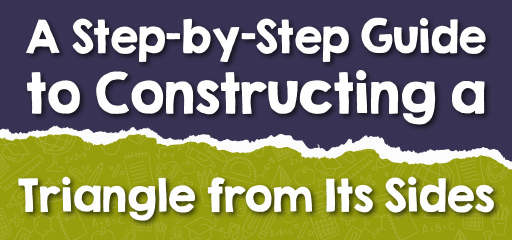# A Step-by-Step Guide to Constructing a Triangle from Its Sides

In geometry, triangles are among the most basic and versatile shapes. Constructing a triangle using its three given sides is a fundamental skill. This guide will walk you through the steps and principles behind this construction, ensuring you can create accurate triangles with ease and precision.## Step-by-step Guide: Constructing a Triangle Given Its Sides

Tools Required:

• A straightedge or ruler.
• A compass.
• A pencil.

Procedure:
i. Laying the Base: Draw one of the triangle’s sides using your straightedge. Let’s name this side $$AB$$.

ii. Constructing the Second Side: Choose one endpoint (let’s say $$A$$). Using your compass, set its width to the length of one of the other sides (let’s say $$AC$$). Then, draw a circular arc with $$A$$ as the center.

iii. Constructing the Third Side: Similarly, set your compass to the length of the third side (let’s say $$BC$$). Place the compass point on $$B$$ and draw another arc that intersects the previous arc.

iv. Finalizing the Triangle: Label the point where the arcs intersect as $$C$$. Use your straightedge to connect points $$C$$ to both $$A$$ and $$B$$. The resulting shape is the desired triangle $$ABC$$.

### Examples

Example 1:
You’re tasked with designing a triangular park, and you have been given the three side lengths but no angle measurements.

Solution:
Utilize the aforementioned method to draw the triangle accurately on a blueprint. Once constructed, you can proceed with additional design and landscaping within this triangular boundary.

Example 2:
You have side lengths $$5 \text{ cm}$$, $$7 \text{ cm}$$, and $$8 \text{ cm}$$. Can a triangle be constructed with these sides?

Solution:
Yes. The sum of any two sides of a triangle (in this case, $$5 + 7 = 12$$ and $$7 + 8 = 15$$) is greater than the third side ($$8 \text{ cm}$$). Thus, a triangle can be constructed using these lengths by following the steps mentioned.

### Practice Questions:

1. What conditions must three side lengths fulfill to construct a triangle?
2. After constructing a triangle using three side lengths, how can you ascertain if it’s a right triangle?
3. Using side lengths $$4 \text{ cm}$$, $$6 \text{ cm}$$, and $$11 \text{ cm}$$, is triangle construction feasible?

1. The sum of any two side lengths must exceed the third. This principle is termed the triangle inequality theorem.
2. Post-construction, apply the Pythagorean theorem to all sides. If the square of the longest side equals the sum of the squares of the other two, it’s a right triangle.
3. No. The combined lengths of the shorter sides, $$4 + 6 = 10 \text{ cm}$$, falls short of the longest side’s length $$11 \text{ cm}$$. Triangle construction isn’t possible with these measurements due to the triangle inequality theorem.

### What people say about "A Step-by-Step Guide to Constructing a Triangle from Its Sides - Effortless Math: We Help Students Learn to LOVE Mathematics"?

No one replied yet.

X
30% OFF

Limited time only!

Save Over 30%

SAVE $5 It was$16.99 now it is \$11.99